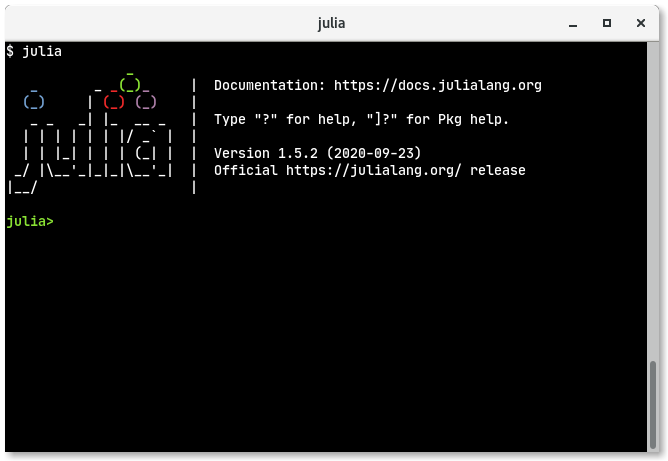REPL stands for Read Eval(uate) Print Loop. Its a way a programming ecosystem gives you a easy way to execute small programs in shell environments. Let’s get started with it. In your terminal type this:

\$ julia


You should be seeing something like this.Juila prints a nice ASCII art of Julia logo and it prints version info and when was it released etc. The julia> is julia prompt where you can type some small Julia code, Julia will read it, evaluate it and print the result.

So lets see what 1 and 2 added is, type 1 + 2 and hit ENTER:

julia> 1 + 2
3


So it’s 3 yaaay! Our first small Julia program!!

You can type multiple Julia statements per line, it needs to be separated by semicolon ; as shown:

julia> x = 3; y = 4
4


In the above statement we have a thing like x = 3, this means we put a value of 3 in a box named x and in y = 4, we put 4 in a box named y. Now what happens when we have three x boxes and four y boxes? Try this out:

julia> 3x + 4y
25


Julia says its 25!

Now let’s try to multiply x and y:

julia> xy
ERROR: UndefVarError: xy not defined


It fails. We will see why it fails when we see about variables. Okay, mathematically we use dot . to denote multiplication, so let’s try out x.y:

julia> x.y
ERROR: type Int64 has no field y
Stacktrace:
 getproperty(::Int64, ::Symbol) at ./Base.jl:33
 top-level scope at REPL:1


it fails too, we will soon see why in coming blogs (i.e you will learn about it). The right way to multiply in Julia is to use the star * operator as shown:

julia> x * y
12


# Volume of Sphere

Le’s see something complex. Let’s now calculate volume of a sphere. We know its $${4 \over 3} \pi r^3$$, lets see how to do it in Julia.

First let’s have a variables name radius to hold value of radius, so let’s say the radius is 7 units, so we use this statement to assign 7 to radius:

julia> radius = 7
7


Next we calculate the volume as follows:

julia> (4/3)π * radius^3
1436.7550402417319


If you are wondering how I got π into Julia REPL, just type \pi and press TAB. Here radius^3 means radius is raised to the power of 3.

Julia provides a function named typeof, with which we can investigate the type of π as follows:

julia> typeof(π)
Irrational{:π}


so Julia says π is irrational which is right.

Rational numbers in Julia can be defined like x//y, so typeof(22//7) returns rational as shown:

julia> typeof(22//7)
Rational{Int64}


# Clearing REPL

When you feel there are lot of things in your Julia REPL and want to have a fresh screen, just type Ctrl + l and it will blank out as shown:You can still access old variables and functions defined, its just the display that’s blanked out.

# Exiting a statement

When you have typed something wrong and want to terminate the statement, just type Ctrl + c

julia> 1 + 41^C

julia>


Julia will clear out that statement and present a fresh prompt.

# History

Use the up arrow and down arrow to go through the history of all the statements you have typed.

# Exiting REPL

To exit Julia REPL press Ctrl + d.

# Conclusion

In this blog I have introduced to Julia REPL. In upcoming blogs will be discussing more of it. Bye.